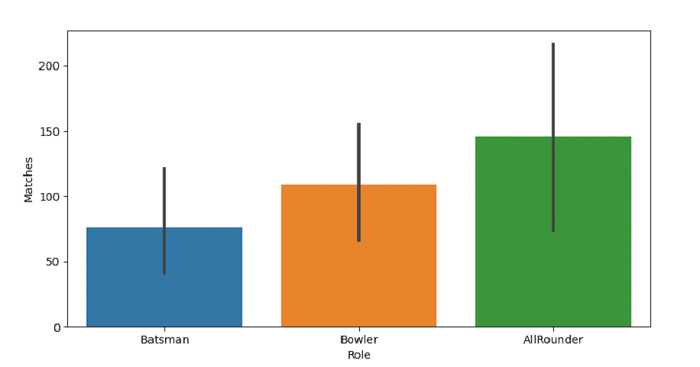Python Pandas - Draw a set of vertical bar plots grouped by a categorical variable with Seaborn

PythonServer Side ProgrammingProgramming

Bar Plot in Seaborn is used to show point estimates and confidence intervals as rectangular bars. The seaborn.barplot() is used for this. Plot vertical bar plots grouped by a categorical variable, by passing the variable as x or y coordinates in the barplot() method.

Let’s say the following is our dataset in the form of a CSV file − Cricketers2.csv

At first, import the required libraries −

import seaborn as sb
import pandas as pd
import matplotlib.pyplot as plt

Load data from a CSV file into a Pandas DataFrame −

Plotting vertical bar plots grouped by a categorical variable −

sb.barplot(x = dataFrame["Role"], y = dataFrame["Matches"])

Example

Following is the code −

import seaborn as sb
import pandas as pd
import matplotlib.pyplot as plt

# Load data from a CSV file into a Pandas DataFrame

# plotting vertical bar plots grouped by a categorical variable
sb.barplot(x = dataFrame["Role"], y = dataFrame["Matches"])

# display
plt.show()

Output

This will produce the following output −